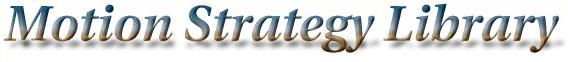Main Page   Class Hierarchy   Compound List   File List   Compound Members   File Members

# Problem Class Reference

An interface class that provides the primary input to a planner. More...

#include <problem.h>

List of all members.

## Public Methods

Problem (Geom *geom, Model *model, string path)
Problem must be given any instance of Geom and any instance of Model from each of their class hierarchies.

virtual ~Problem ()
Empty destructor.

void SetGeom (Geom *geom)
Change the instance of Geom.

void SetModel (Model *model)
Change the instance of Model.

virtual list<MSLVectorGetInputs (const MSLVector &x)
Return a list of possible inputs, which may depend on state.

virtual list<MSLVectorGetInputs ()
Return a list of possible inputs.

virtual MSLVector Integrate (const MSLVector &x, const MSLVector &u, const double &deltat)
Perform integration from state x, using input u, over time deltat.

virtual MSLVector InterpolateState (const MSLVector &x1, const MSLVector &x2, const double &a)
Linearly interpolate two states while respecting topology. More...

virtual double Metric (const MSLVector &x1, const MSLVector &x2)
A distance metric defined in Model.

virtual MSLVector StateToConfiguration (const MSLVector &x)
A method that converts a Model state in to a Geom configuration.

virtual MSLVector StateDifference (const MSLVector &x1, const MSLVector &x2)
Compute a MSLVector based on x2-x1. In R^n, the states are simply subtracted to make the MSLVector. This method exists to make things work correctly for other state-space topologies.

virtual bool Satisfied (const MSLVector &x)
This takes the logical AND of CollisionFree from Geom, and Satisfied from Model.

virtual bool CollisionFree (const MSLVector &q)
The collision checker passed in from Geom.

virtual double DistanceComp (const MSLVector &q)
The distance computation algorithm from Geom.

virtual MSLVector ConfigurationDifference (const MSLVector &q1, const MSLVector &q2)
Compute a MSLVector based on q2-q1. In R^n, the configurations are simply subtracted to make the MSLVector. This method exists to make things work correctly for other configuration-space topologies.

## Public Attributes

string FilePath
The directory in which all files for a problem will be stored.

int NumBodies
The number of rigid bodies.

int StateDim
The dimenson of the state space.

int InputDim
The dimension of the input space.

int GeomDim
The dimension of the geometric model.

MSLVector LowerState
MSLVector of minimum values for each state variable.

MSLVector UpperState
MSLVector of maximum values for each state variable.

MSLVector InitialState
The starting state for a planner.

MSLVector GoalState
The goal state for a planner.

MSLVector MaxDeviates
Maximum displacement of geometry with respect to change in each variable.

## Protected Attributes

GeomG
Need to define a geometry for collision detection.

ModelM
xdot = f(x,u), integration technique, state bounds.

## Detailed Description

An interface class that provides the primary input to a planner.

This interface class contains protected instances of Geom and Model. Wrappers to methods from Geom provide collision detection and distance computation. Wrappers to methods from Model provide incremental simulation of a kinematic or dynamical system. It is expected a planner can get all (or nearly all) of the information it needs from Problem.

## Constructor & Destructor Documentation

 Problem::Problem ( Geom * geom, Model * model, string path )
 Problem must be given any instance of Geom and any instance of Model from each of their class hierarchies.

 Problem::~Problem ( ) [inline, virtual]
 Empty destructor.

## Member Function Documentation

 bool Problem::CollisionFree ( const MSLVector & q ) [virtual]
 The collision checker passed in from Geom.

 MSLVector Problem::ConfigurationDifference ( const MSLVector & q1, const MSLVector & q2 ) [virtual]
 Compute a MSLVector based on q2-q1. In R^n, the configurations are simply subtracted to make the MSLVector. This method exists to make things work correctly for other configuration-space topologies.

 double Problem::DistanceComp ( const MSLVector & q ) [virtual]
 The distance computation algorithm from Geom.

 list< MSLVector > Problem::GetInputs ( ) [virtual]
 Return a list of possible inputs.

 list< MSLVector > Problem::GetInputs ( const MSLVector & x ) [virtual]
 Return a list of possible inputs, which may depend on state.

 MSLVector Problem::Integrate ( const MSLVector & x, const MSLVector & u, const double & deltat ) [virtual]
 Perform integration from state x, using input u, over time deltat.

 MSLVector Problem::InterpolateState ( const MSLVector & x1, const MSLVector & x2, const double & a ) [virtual]
 Linearly interpolate two states while respecting topology. If a=0, then x1 is returned; if a=1, then x2 is returned. All intermediate values of $a \in [0,1]$ yield intermediate states. This method is defined by Model.

 double Problem::Metric ( const MSLVector & x1, const MSLVector & x2 ) [virtual]
 A distance metric defined in Model.

 bool Problem::Satisfied ( const MSLVector & x ) [virtual]
 This takes the logical AND of CollisionFree from Geom, and Satisfied from Model.

 void Problem::SetGeom ( Geom * geom )
 Change the instance of Geom.

 void Problem::SetModel ( Model * model )
 Change the instance of Model.

 MSLVector Problem::StateDifference ( const MSLVector & x1, const MSLVector & x2 ) [virtual]
 Compute a MSLVector based on x2-x1. In R^n, the states are simply subtracted to make the MSLVector. This method exists to make things work correctly for other state-space topologies.

 MSLVector Problem::StateToConfiguration ( const MSLVector & x ) [virtual]
 A method that converts a Model state in to a Geom configuration.

## Member Data Documentation

 string Problem::FilePath
 The directory in which all files for a problem will be stored.

 Geom * Problem::G [protected]
 Need to define a geometry for collision detection.

 int Problem::GeomDim
 The dimension of the geometric model.

 MSLVector Problem::GoalState
 The goal state for a planner.

 MSLVector Problem::InitialState
 The starting state for a planner.

 int Problem::InputDim
 The dimension of the input space.

 MSLVector Problem::LowerState
 MSLVector of minimum values for each state variable.

 Model * Problem::M [protected]
 xdot = f(x,u), integration technique, state bounds.

 MSLVector Problem::MaxDeviates
 Maximum displacement of geometry with respect to change in each variable.

 int Problem::NumBodies
 The number of rigid bodies.

 int Problem::StateDim
 The dimenson of the state space.

 MSLVector Problem::UpperState
 MSLVector of maximum values for each state variable.

The documentation for this class was generated from the following file: Motion Strategy Library

Web page maintained by Steve LaValle
Partial support provided by NSF CAREER Award IRI-970228 (LaValle), Honda Research.
Contributors: Anna Atramentov, Peng Cheng, James Kuffner, Steve LaValle, and Libo Yang.